# 100mA Ammeter using AVR Microcontroller

Published  October 16, 2015   3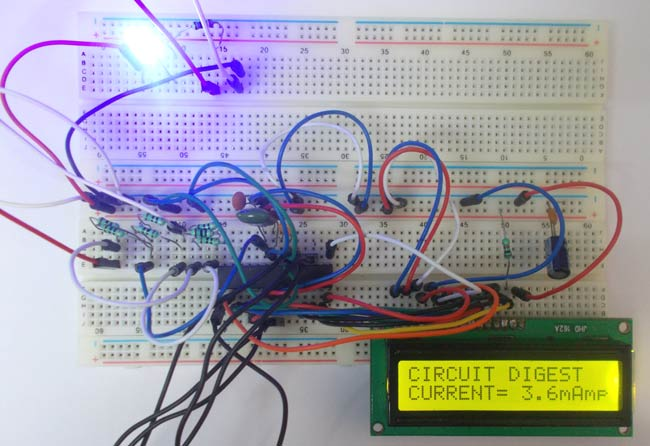In this project we are going to make a low range ammeter using ATMEGA8 microcontroller. In ATMEGA8, we are going use 10bit ADC (Analog to Digital Conversion) feature to do this. Although we have few other ways to get the current parameter from a circuit, we are going to use resistive drop method, because it’s the easiest and simplest way to get current parameter.

In this method we are going to pass the current which needed to be measured in to a small resistance, by this we get a drop across that resistance which is related to current flowing through it. This voltage across resistance is fed to ATMEGA8 for ADC conversion. With that we will have the current in digital value which will be displayed on a 16x2 LCD.

For that we are going to use a voltage divider circuit. We are going to fed the current through the complete resistance branch. The midpoint of branch is taken to measurement. When current changes there will be drop change in the resistance which is linear to it. So with this we have a voltage which changes with linearity.

Now important thing to note here is, the input taken by the controller for ADC conversion is as low as 50µAmp. This loading effect of resistance based voltage divider is important as the current drawn from Vout of voltage divider increases the error percentage increases, for now we need not worry about loading effect.

### Components Required

Hardware: ATMEGA8, power supply (5v), AVR-ISP PROGRAMMER, JHD_162ALCD (16*2LCD), 100uF capacitor, 100nF capacitor (4 pieces), 100Ω resistor (7 pieces) or 2.5Ω (2 pieces), 100KΩ resistor.

Software: Atmel studio 6.1, progisp or flash magic.

### Circuit Diagram and Working Explanation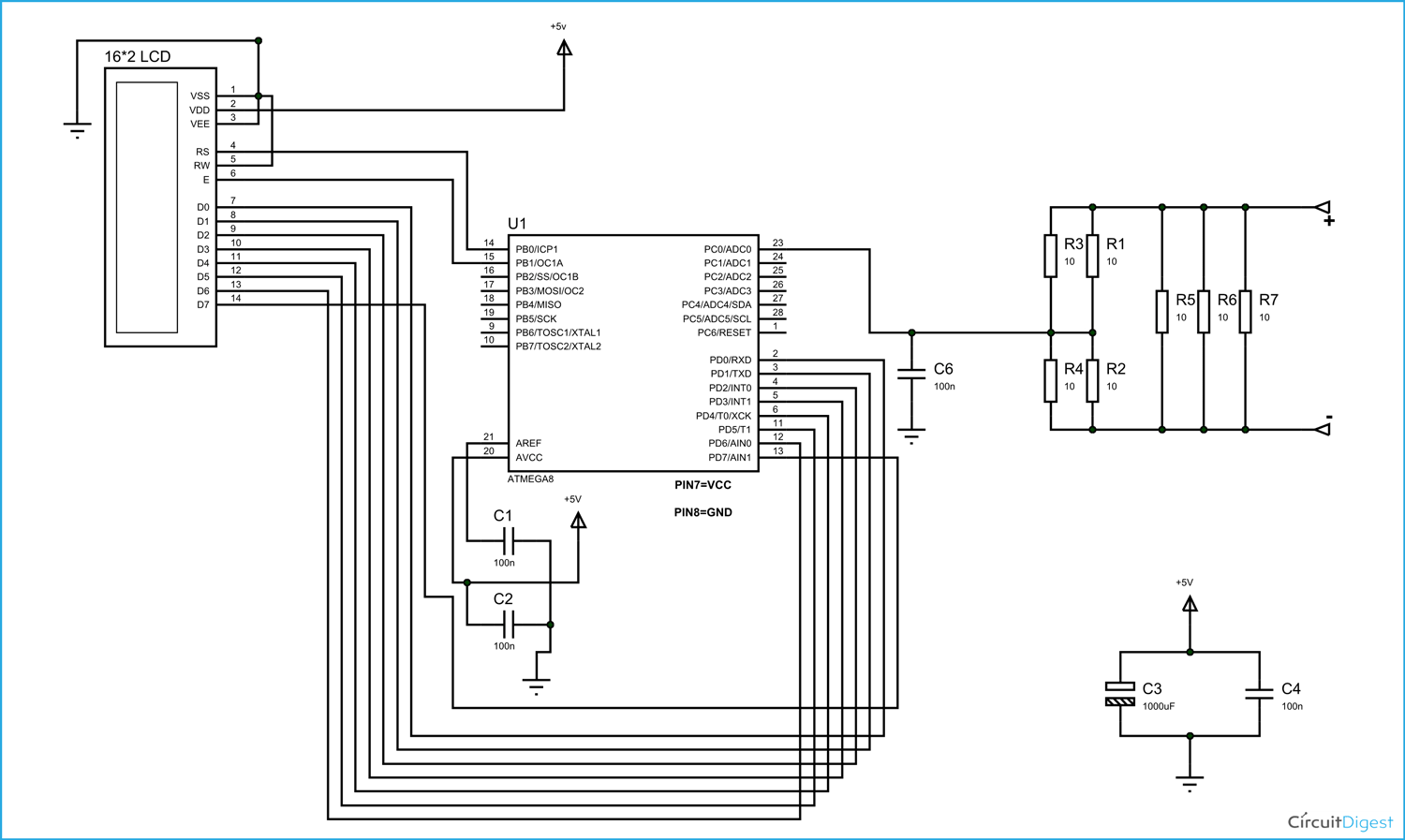[See this tutorial to understand how to interface LCD with AVR Microcontroller]

The voltage across R2 and R4 is not completely linear; it will be a noisy one. To filter out the noise, capacitors are placed across each resistor in the divider circuit as shown in figure.

In ATMEGA8, we can give Analog input to any of FOUR channels of PORTC, it doesn’t matter which channel we choose as all are same. We are going to choose channel 0 or PIN0 of PORTC. In ATMEGA8, the ADC is of 10 bit resolution, so the controller can detect a minimum change of Vref/2^10, so if the reference voltage is 5V we get a digital output increment for every 5/2^10 = 5mV. So for every 5mV increment in the input we will have a increment of one at digital output.

Now we need to set the register of ADC based on the following terms:

1. First of all we need to enable the ADC feature in ADC.

2. Here are going to get a maximum input voltage for ADC conversion is +5V. So we can set up maximum value or reference of ADC to 5V.

3. The controller has a trigger conversion feature that means ADC conversion takes place only after an external trigger, since we don’t want that we need to set the registers for the ADC to run in continuous free running mode.

4. For any ADC, frequency of conversion (Analog value to Digital value) and accuracy of digital output are inversely proportional. So for better accuracy of digital output we have to choose lesser frequency. For normal ADC clock we are setting the presale of ADC to maximum value (2). Since we are using the internal clock of 1MHZ, the clock of ADC will be (1000000/2).

These are the only four things we need to know to getting started with ADC.

All the above four features are set by two registers,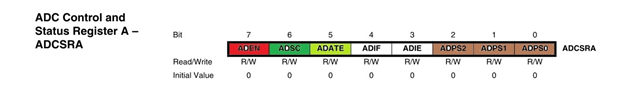RED (ADEN): This bit has to be set for enabling the ADC feature of ATMEGA.

BLUE(REFS1,REFS0): These two bits are used to set the reference voltage (or max input voltage we are going to give).  Since we want to have reference voltage 5V, REFS0 should be set, by the table.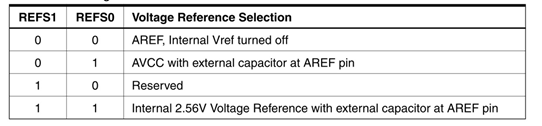YELLOW (ADFR): This bit must be set for the ADC to run continuously (free running mode).

PINK (MUX0-MUX3): These four bits are for telling the input channel. Since we are going to use ADC0 or PIN0, we need not set any bits as by the table.BROWN (ADPS0-ADPS2): these three bits are for setting the prescalar for ADC. Since we are using a prescalar of 2, we have to set one bit.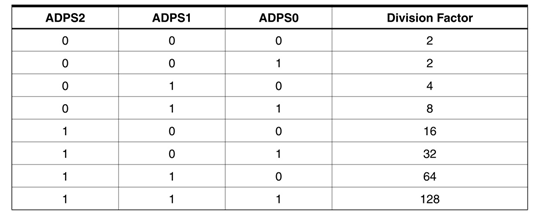DARK GREEN (ADSC): this bit set for the ADC to start conversion. This bit can be disabled in the program when we need to stop the conversion.

[Also check : Digital Voltmeter using AVR Microcontroller]

Code

#include <avr/io.h>
#define F_CPU 1000000
#include <util/delay.h>
#include <stdlib.h>

#define enable            1
#define registerselection 0

void send_a_command(unsigned char command);
void send_a_character(unsigned char character);
void send_a_string(char *string_of_characters);

int main(void)
{
DDRD = 0xFF;
DDRC = 0;
DDRB= 0xFF;

float i =0;
float CURRENT = 0;
char CURRENTSHOW ;

send_a_command(0x01); //Clear Screen 0x01 = 00000001
_delay_ms(5);
send_a_command(0x38);
_delay_ms(5);
send_a_command(0b00001111);
_delay_ms(5);

send_a_string ("CIRCUIT DIGEST  ");
send_a_command(0x80 + 0x40 + 0);
send_a_string ("CURRENT=");
send_a_command(0x80 + 0x40 + 8);

while(1)
{
dtostrf(CURRENT, 4, 1, CURRENTSHOW);
send_a_string(CURRENTSHOW);
send_a_string("mAmp");
_delay_ms(50);
send_a_command(0x80 + 0x40 + 8);

//dtostr(double precision value, width, precision, string that will store the numbers);
// Value is either a direct value plugged into this place, or a variable to contains a value.
//Width that is used with dtostrf is the number of characters in the number that includes the negative sign (-). For instance, if the number is -532.87, the width would be 7 including the negative sign and the decimal point.
//Precision is how many numbers would be after the decimal point in the dtostrf usage.
//Base is the maximum number of values per digit. For instance, 2 is for binary representation (2 possible values for each digit - 0 or 1); 10 is for the common human number system (10 possible values for each digit - 0, 1, 2, 3, 4, 5, 6, 7, 8, or 9); 16 is for hexadecimal where there are 16 possible values for each digit - 0, 1, 2, 3, 4, 5, 6, 7, 8, 9, A, B, C, D, E, or F. It should be understood that if the base is larger, then the alphabet will just get larger.
//String is the variable that accepts alphanumeric characters such as a char array. After the function is executed, the string will have the number stored within the array. For instance, if an integer variable contains the number 643, then the string variable will contain "634".

}
}

void send_a_command(unsigned char command)
{
PORTD = command;
PORTB &= ~ (1<<registerselection);
PORTB |= 1<<enable;
_delay_ms(2);
PORTB &= ~1<<enable;
PORTD = 0;
}

void send_a_character(unsigned char character)
{
PORTD = character;
PORTB |= 1<<registerselection;
PORTB |= 1<<enable;
_delay_ms(2);
PORTB &= ~1<<enable;
PORTD = 0;
}
void send_a_string(char *string_of_characters)
{
while(*string_of_characters > 0)
{
send_a_character(*string_of_characters++);
}
}

Video

Tags

Submitted by dominic on Fri, 12/23/2016 - 16:42

### what are the fusebits

what are the fusebits

Submitted by Alien on Wed, 09/20/2017 - 21:10